# Tag: Grothendieck

I’m on vacation, and re-reading two ‘metabiographies’:

and

Siobhan Roberts : Genius At Play: The Curious Mind of John Horton Conway.

Siobhan Roberts’ book is absolutely brilliant! I’m reading it for the n-th time, first on Kindle, then hardcopy, and now I’m just flicking through its pages, whenever I want to put a smile on my face.

So, here’s today’s gem of a Conway quote (on page 150):

Pure mathematicians usually don’t found companies and deal with the world in an aggressive way. We sit in our ivory towers and think.

(Conway complains his words were taken out of context, in an article
featuring Stephen Wolfram.)

If only university administrations worldwide would accept the ‘sitting in an ivory tower and think’-bit as the job description, and evaluation criterium, for their pure mathematicians.

Sadly… they prefer managers to thinkers.

This reminds me of another brilliant text, perhaps not receiving the attention it deserves:

Daniel J. Woodhouse : An open letter to the mathematical community.

Woodhouse offers a reaction to the ‘neoliberal upper management and bloated administration’ of universities:

Within the sphere of pure mathematics — the oldest and most successful of humanity’s intellectual endeavors — I believe our best chance at preserving the integrity and dignity of our tradition is to return to our Pythagorean roots. We should become a cult.

How?

Let us seclude ourselves in mountain caves and daub mysterious equations in blood across rock-faces to ward off outsiders. Let us embrace our most impenetrable mathematical texts as sacred and requiring divinely distributed revelation.

Why?

I am convinced that the current system has dulled our understanding of the value we offer through our instruction. Modern mathematical techniques are the foundation of modern science, medicine, and technology, and we should be the literal, rather than metaphorical, high priests of this temple. Only by withholding our insights will we be able to reassert the intrinsic worth of our knowledge.

I hope these few paragraphs have wetted your appetite to read the manifesto in full, and then take action!

I’m trying to get into the latest Manin-Marcolli paper Quantum Statistical Mechanics of the Absolute Galois Group on how to create from Grothendieck’s dessins d’enfant a quantum system, generalising the Bost-Connes system to the non-Abelian part of the absolute Galois group $Gal(\overline{\mathbb{Q}}/\mathbb{Q})$.

In doing so they want to extend the action of the multiplicative monoid $\mathbb{N}_{\times}$ by power maps on the roots of unity to the action of a larger monoid on all dessins d’enfants.

Here they use an idea, originally due to Jordan Ellenberg, worked out by Melanie Wood in her paper Belyi-extending maps and the Galois action on dessins d’enfants.To grasp this, it’s best to remember what dessins have to do with Belyi maps, which are maps defined over $\overline{\mathbb{Q}}$
$\pi : \Sigma \rightarrow \mathbb{P}^1$
from a Riemann surface $\Sigma$ to the complex projective line (aka the 2-sphere), ramified only in $0,1$ and $\infty$. The dessin determining $\pi$ is the 2-coloured graph on the surface $\Sigma$ with as black vertices the pre-images of $0$, white vertices the pre-images of $1$ and these vertices are joined by the lifts of the closed interval $[0,1]$, so the number of edges is equal to the degree $d$ of the map.

Wood considers a very special subclass of these maps, which she calls Belyi-extender maps, of the form
$\gamma : \mathbb{P}^1 \rightarrow \mathbb{P}^1$
defined over $\mathbb{Q}$ with the additional property that $\gamma$ maps $\{ 0,1,\infty \}$ into $\{ 0,1,\infty \}$.

The upshot being that post-compositions of Belyi’s with Belyi-extenders $\gamma \circ \pi$ are again Belyi maps, and if two Belyi’s $\pi$ and $\pi’$ lie in the same Galois orbit, then so must all $\gamma \circ \pi$ and $\gamma \circ \pi’$.

The crucial Ellenberg-Wood idea is then to construct “new Galois invariants” of dessins by checking existing and easily computable Galois invariants on the dessins of the Belyi’s $\gamma \circ \pi$.

For this we need to know how to draw the dessin of $\gamma \circ \pi$ on $\Sigma$ if we know the dessins of $\pi$ and of the Belyi-extender $\gamma$. Here’s the procedure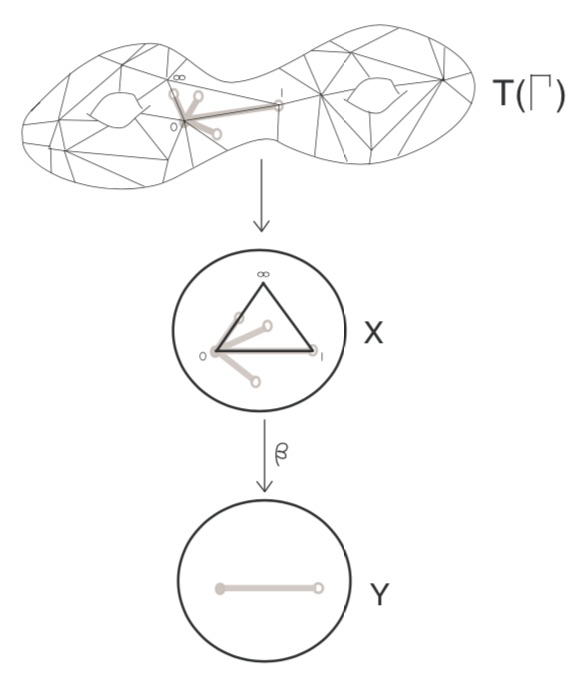Here, the middle dessin is that of the Belyi-extender $\gamma$ (which in this case is the power map $t \rightarrow t^4$) and the upper graph is the unmarked dessin of $\pi$.

One has to replace each of the black-white edges in the dessin of $\pi$ by the dessin of the expander $\gamma$, but one must be very careful in respecting the orientations on the two dessins. In the upper picture just one edge is replaced and one has to do this for all edges in a compatible manner.

Thus, a Belyi-expander $\gamma$ inflates the dessin $\pi$ with factor the degree of $\gamma$. For this reason i prefer to call them dessinflateurs, a contraction of dessin+inflator.

In her paper, Melanie Wood says she can separate dessins for which all known Galois invariants were the same, such as these two dessins,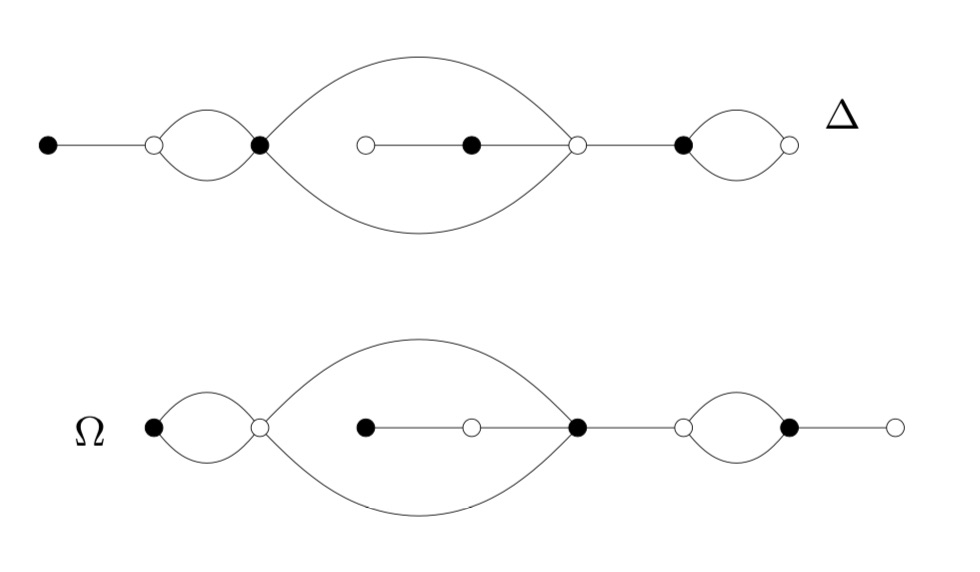by inflating them with a suitable Belyi-extender and computing the monodromy group of the inflated dessin.

This monodromy group is the permutation group generated by two elements, the first one gives the permutation on the edges given by walking counter-clockwise around all black vertices, the second by walking around all white vertices.

For example, by labelling the edges of $\Delta$, its monodromy is generated by the permutations $(2,3,5,4)(1,6)(8,10,9)$ and $(1,3,2)(4,7,5,8)(9,10)$ and GAP tells us that the order of this group is $1814400$. For $\Omega$ the generating permutations are $(1,2)(3,6,4,7)(8,9,10)$ and $(1,2,4,3)(5,6)(7,9,8)$, giving an isomorphic group.

Let’s inflate these dessins using the Belyi-extender $\gamma(t) = -\frac{27}{4}(t^3-t^2)$ with corresponding dessin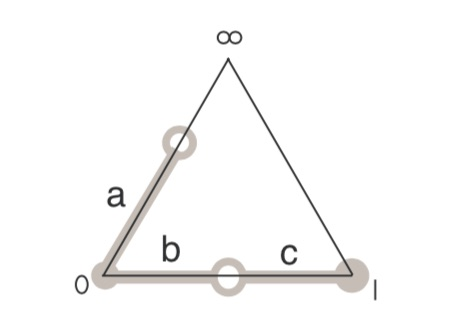It took me a couple of attempts before I got the inflated dessins correct (as i knew from Wood that this simple extender would not separate the dessins). Inflated $\Omega$ on top: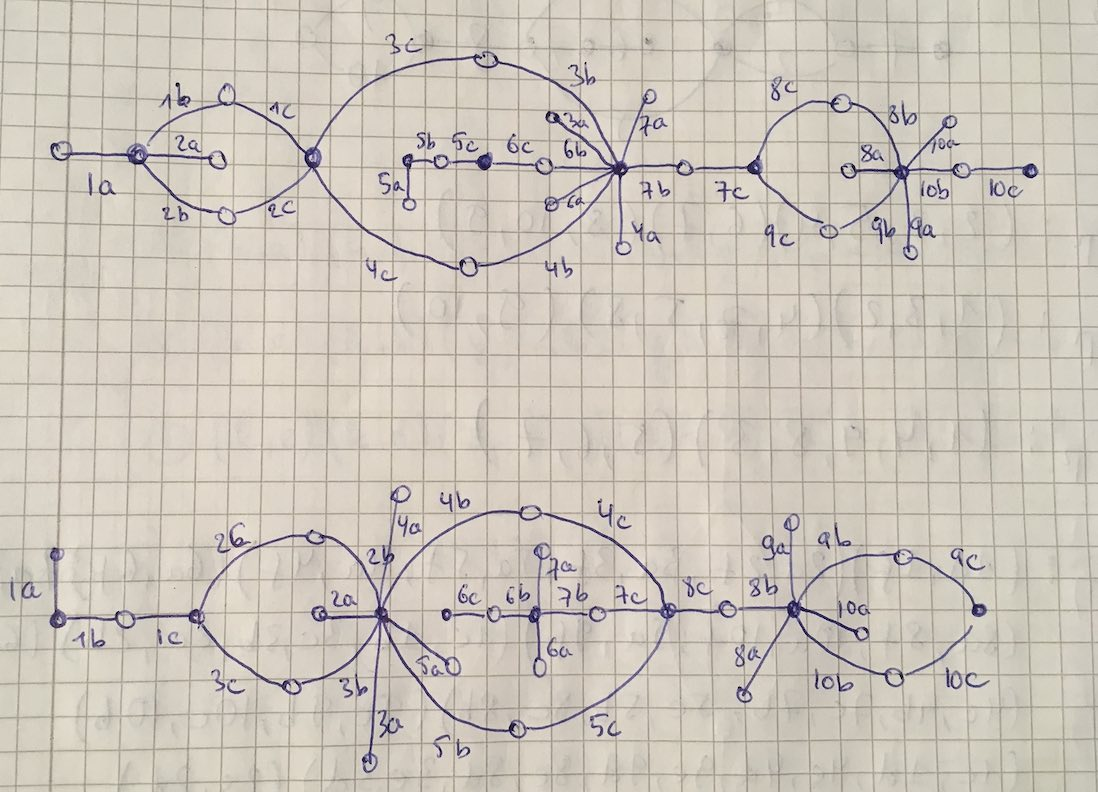Both dessins give a monodromy group of order $35838544379904000000$.

Now we’re ready to do serious work.

Melanie Wood uses in her paper the extender $\zeta(t)=\frac{27 t^2(t-1)^2}{4(t^2-t+1)^3}$ with associated dessin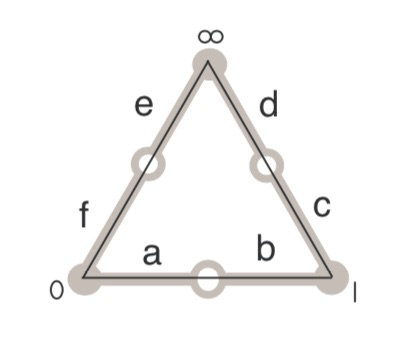and says she can now separate the inflated dessins by the order of their monodromy groups. She gets for the inflated $\Delta$ the order $19752284160000$ and for inflated $\Omega$ the order $214066877211724763979841536000000000000$.

It’s very easy to make mistakes in these computations, so probably I did something horribly wrong but I get for both $\Delta$ and $\Omega$ that the order of the monodromy group of the inflated dessin is $214066877211724763979841536000000000000$.

I’d be very happy when someone would be able to spot the error!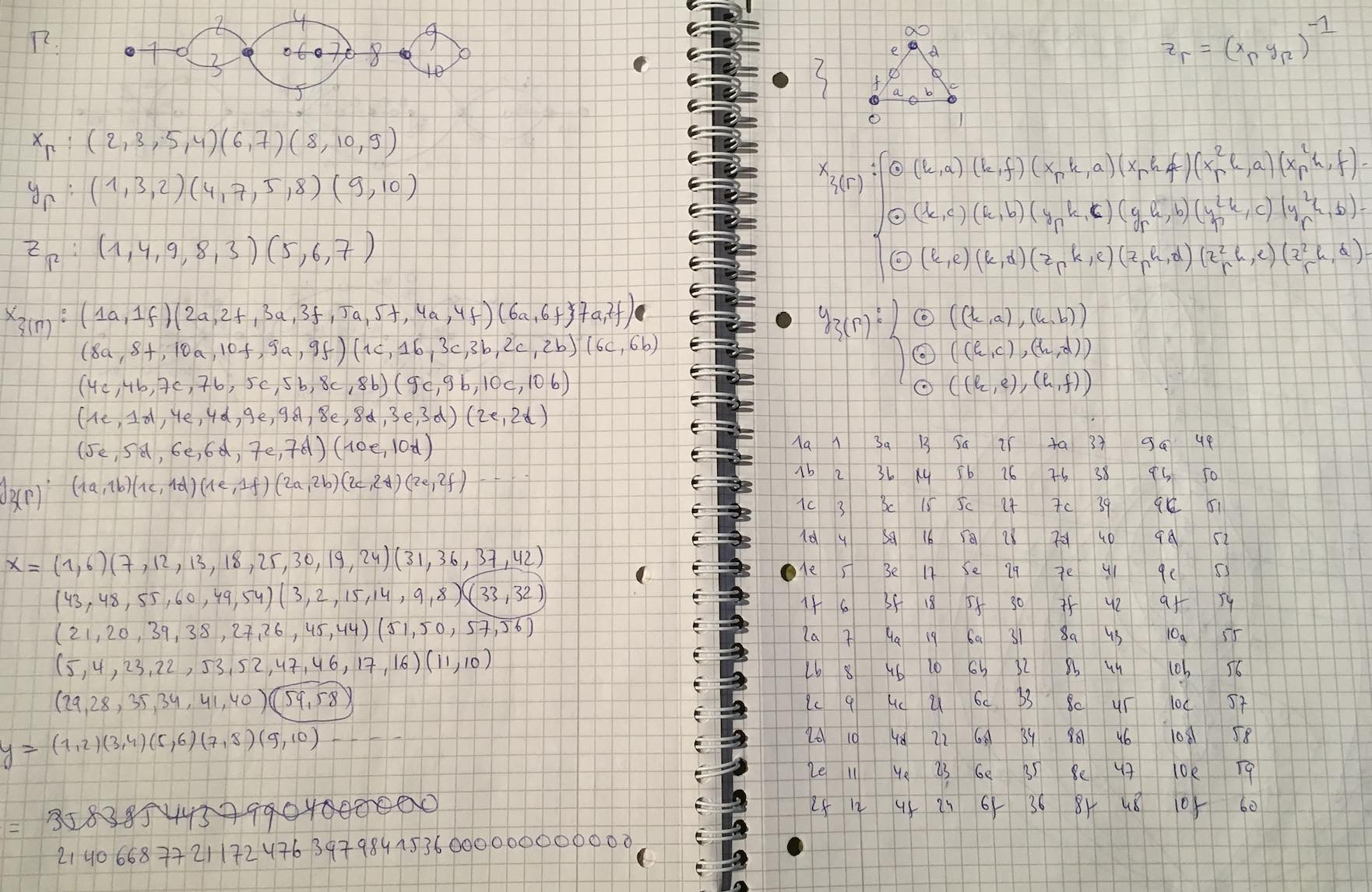Dedekind’s Psi-function $\Psi(n)= n \prod_{p |n}(1 + \frac{1}{p})$ pops up in a number of topics:

• $\Psi(n)$ is the index of the congruence subgroup $\Gamma_0(n)$ in the modular group $\Gamma=PSL_2(\mathbb{Z})$,
• $\Psi(n)$ is the number of points in the projective line $\mathbb{P}^1(\mathbb{Z}/n\mathbb{Z})$,
• $\Psi(n)$ is the number of classes of $2$-dimensional lattices $L_{M \frac{g}{h}}$ at hyperdistance $n$ in Conway’s big picture from the standard lattice $L_1$,
• $\Psi(n)$ is the number of admissible maximal commuting sets of operators in the Pauli group of a single qudit.

The first and third interpretation have obvious connections with Monstrous Moonshine.

Conway’s big picture originated from the desire to better understand the Moonshine groups, and Ogg’s Jack Daniels problem
asks for a conceptual interpretation of the fact that the prime numbers such that $\Gamma_0(p)^+$ is a genus zero group are exactly the prime divisors of the order of the Monster simple group.

Here’s a nice talk by Ken Ono : Can’t you just feel the Moonshine?

For this reason it might be worthwhile to make the connection between these two concepts and the number of points of $\mathbb{P}^1(\mathbb{Z}/n\mathbb{Z})$ as explicit as possible.

Surely all of this is classical, but it is nicely summarised in the paper by Tatitscheff, He and McKay “Cusps, congruence groups and monstrous dessins”.

The ‘monstrous dessins’ from their title refers to the fact that the lattices $L_{M \frac{g}{h}}$ at hyperdistance $n$ from $L_1$ are permuted by the action of the modular groups and so determine a Grothendieck’s dessin d’enfant. In this paper they describe the dessins corresponding to the $15$ genus zero congruence subgroups $\Gamma_0(n)$, that is when $n=1,2,3,4,5,6,7,8,9,10,12,13,16,18$ or $25$.

Here’s the ‘monstrous dessin’ for $\Gamma_0(6)$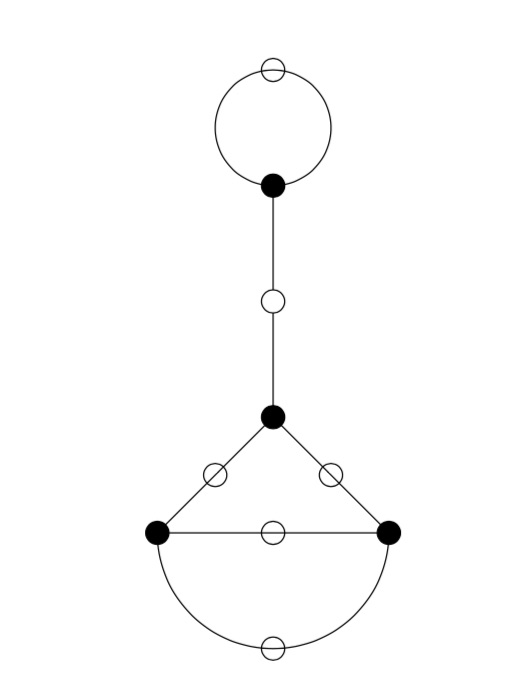But, one can compute these dessins for arbitrary $n$, describing the ripples in Conway’s big picture, and try to figure out whether they are consistent with the Riemann hypothesis.

We will get there eventually, but let’s start at an easy pace and try to describe the points of the projective line $\mathbb{P}^1(\mathbb{Z}/n \mathbb{Z})$.

Over a field $k$ the points of $\mathbb{P}^1(k)$ correspond to the lines through the origin in the affine plane $\mathbb{A}^2(k)$ and they can represented by projective coordinates $[a:b]$ which are equivalence classes of couples $(a,b) \in k^2- \{ (0,0) \}$ under scalar multiplication with non-zero elements in $k$, so with points $[a:1]$ for all $a \in k$ together with the point at infinity $[1:0]$. When $n=p$ is a prime number we have $\# \mathbb{P}^1(\mathbb{Z}/p\mathbb{Z}) = p+1$. Here are the $8$ lines through the origin in $\mathbb{A}^2(\mathbb{Z}/7\mathbb{Z})$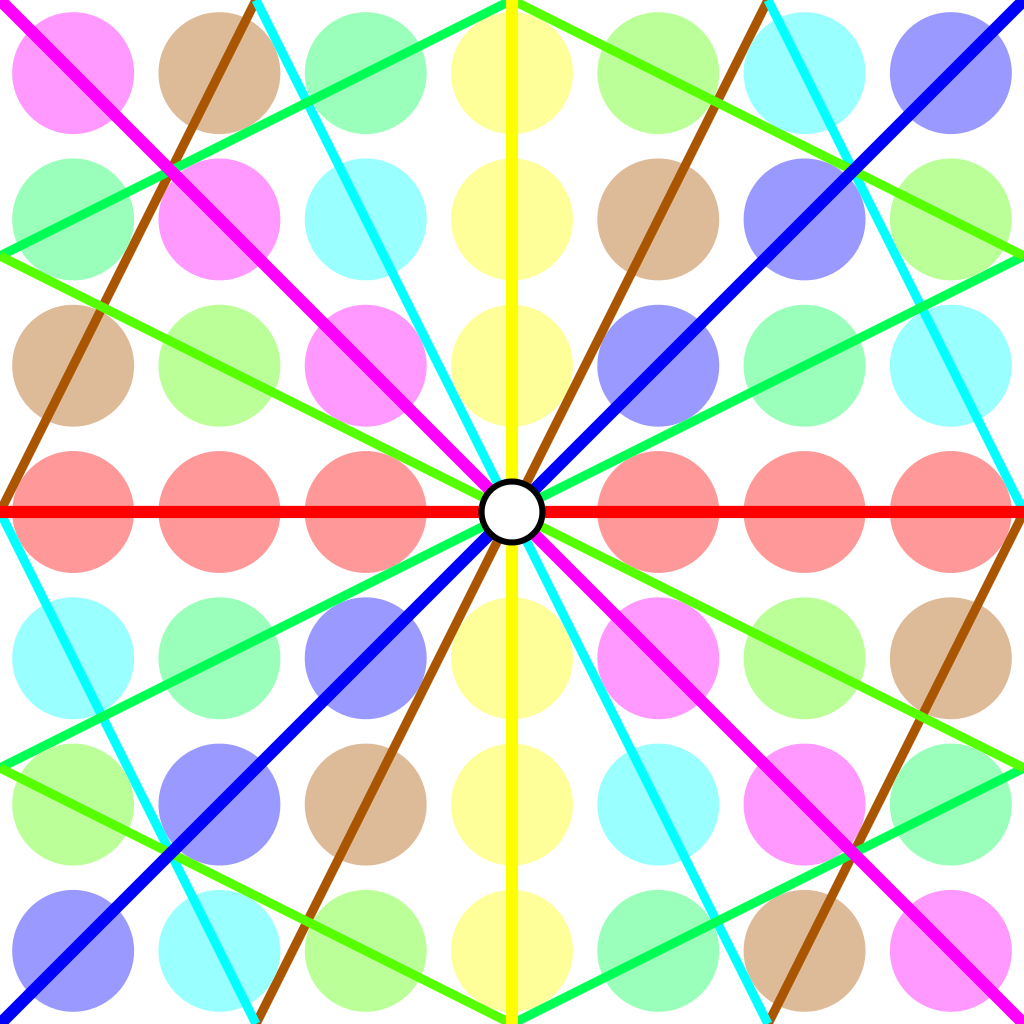Over an arbitrary (commutative) ring $R$ the points of $\mathbb{P}^1(R)$ again represent equivalence classes, this time of pairs
$(a,b) \in R^2~:~aR+bR=R$
with respect to scalar multiplication by units in $R$, that is
$(a,b) \sim (c,d)~\quad~\text{iff}~\qquad \exists \lambda \in R^*~:~a=\lambda c, b = \lambda d$
For $\mathbb{P}^1(\mathbb{Z}/n \mathbb{Z})$ we have to find all pairs of integers $(a,b) \in \mathbb{Z}^2$ with $0 \leq a,b < n$ with $gcd(a,b)=1$ and use Cremona’s trick to test for equivalence:
$(a,b) = (c,d) \in \mathbb{P}^1(\mathbb{Z}/n \mathbb{Z})~\quad \text{iff}~\quad ad-bc \equiv 0~mod~n$
The problem is to find a canonical representative in each class in an efficient way because this is used a huge number of times in working with modular symbols.

Perhaps the best algorithm, for large $n$, is sketched in pages 145-146 of Bill Stein’s Modular forms: a computational approach.

For small $n$ the algorithm in $\S 1.3$ in the Tatitscheff, He and McKay paper suffices:

• Consider the action of $(\mathbb{Z}/n\mathbb{Z})^*$ on $\{ 0,1,…,n-1 \}=\mathbb{Z}/n\mathbb{Z}$ and let $D$ be the set of the smallest elements in each orbit,
• For each $d \in D$ compute the stabilizer subgroup $G_d$ for this action and let $C_d$ be the set of smallest elements in each $G_d$-orbit on the set of all elements in $\mathbb{Z}/n \mathbb{Z}$ coprime with $d$,
• Then $\mathbb{P}^1(\mathbb{Z}/n\mathbb{Z})= \{ [c:d]~|~d \in D, c \in C_d \}$.

Let’s work this out for $n=12$ which will be our running example (the smallest non-squarefree non-primepower):

• $(\mathbb{Z}/12\mathbb{Z})^* = \{ 1,5,7,11 \} \simeq C_2 \times C_2$,
• The orbits on $\{ 0,1,…,11 \}$ are
$\{ 0 \}, \{ 1,5,7,11 \}, \{ 2,10 \}, \{ 3,9 \}, \{ 4,8 \}, \{ 6 \}$
and $D=\{ 0,1,2,3,4,6 \}$,
• $G_0 = C_2 \times C_2$, $G_1 = \{ 1 \}$, $G_2 = \{ 1,7 \}$, $G_3 = \{ 1,5 \}$, $G_4=\{ 1,7 \}$ and $G_6=C_2 \times C_2$,
• $1$ is the only number coprime with $0$, giving us $[1:0]$,
• $\{ 0,1,…,11 \}$ are all coprime with $1$, and we have trivial stabilizer, giving us the points $[0:1],[1:1],…,[11:1]$,
• $\{ 1,3,5,7,9,11 \}$ are coprime with $2$ and under the action of $\{ 1,7 \}$ they split into the orbits
$\{ 1,7 \},~\{ 3,9 \},~\{ 5,11 \}$
giving us the points $[1:2],[3:2]$ and $[5:2]$,
• $\{ 1,2,4,5,7,8,10,11 \}$ are coprime with $3$, the action of $\{ 1,5 \}$ gives us the orbits
$\{ 1,5 \},~\{ 2,10 \},~\{ 4,8 \},~\{ 7,11 \}$
and additional points $[1:3],[2:3],[4:3]$ and $[7:3]$,
• $\{ 1,3,5,7,9,11 \}$ are coprime with $4$ and under the action of $\{ 1,7 \}$ we get orbits
$\{ 1,7 \},~\{ 3,9 \},~\{ 5,11 \}$
and points $[1:4],[3:4]$ and $[5,4]$,
• Finally, $\{ 1,5,7,11 \}$ are the only coprimes with $6$ and they form a single orbit under $C_2 \times C_2$ giving us just one additional point $[1:6]$.

This gives us all $24= \Psi(12)$ points of $\mathbb{P}^1(\mathbb{Z}/12 \mathbb{Z})$ (strangely, op page 43 of the T-H-M paper they use different representants).

One way to see that $\# \mathbb{P}^1(\mathbb{Z}/n \mathbb{Z}) = \Psi(n)$ comes from a consequence of the Chinese Remainder Theorem that for the prime factorization $n = p_1^{e_1} … p_k^{e_k}$ we have
$\mathbb{P}^1(\mathbb{Z}/n \mathbb{Z}) = \mathbb{P}^1(\mathbb{Z}/p_1^{e_1} \mathbb{Z}) \times … \times \mathbb{P}^1(\mathbb{Z}/p_k^{e_k} \mathbb{Z})$
and for a prime power $p^k$ we have canonical representants for $\mathbb{P}^1(\mathbb{Z}/p^k \mathbb{Z})$
$[a:1]~\text{for}~a=0,1,…,p^k-1~\quad \text{and} \quad [1:b]~\text{for}~b=0,p,2p,3p,…,p^k-p$
which shows that $\# \mathbb{P}^1(\mathbb{Z}/p^k \mathbb{Z}) = (p+1)p^{k-1}= \Psi(p^k)$.

Next time, we’ll connect $\mathbb{P}^1(\mathbb{Z}/n \mathbb{Z})$ to Conway’s big picture and the congruence subgroup $\Gamma_0(n)$.

Fortunately, there are a few certainties left in life:

In spring, you might expect the next instalment of Connes’ and Consani’s quest for Gabriel’s topos. Here’s the latest: $\overline{\mathbf{Spec}(\mathbb{Z})}$ and the Gromov norm.

Every half year or so, Mochizuki’s circle-of-friends tries to create some buzz announcing the next IUTeich-workshop. I’ll spare you the link, if you are still interested, follow math_jin or IUTT_bot_math_jin on Twitter.

And then, there’s the never-ending story of Grothendieck’s griboullis, kept alive by the French journalist and author Philippe Douroux.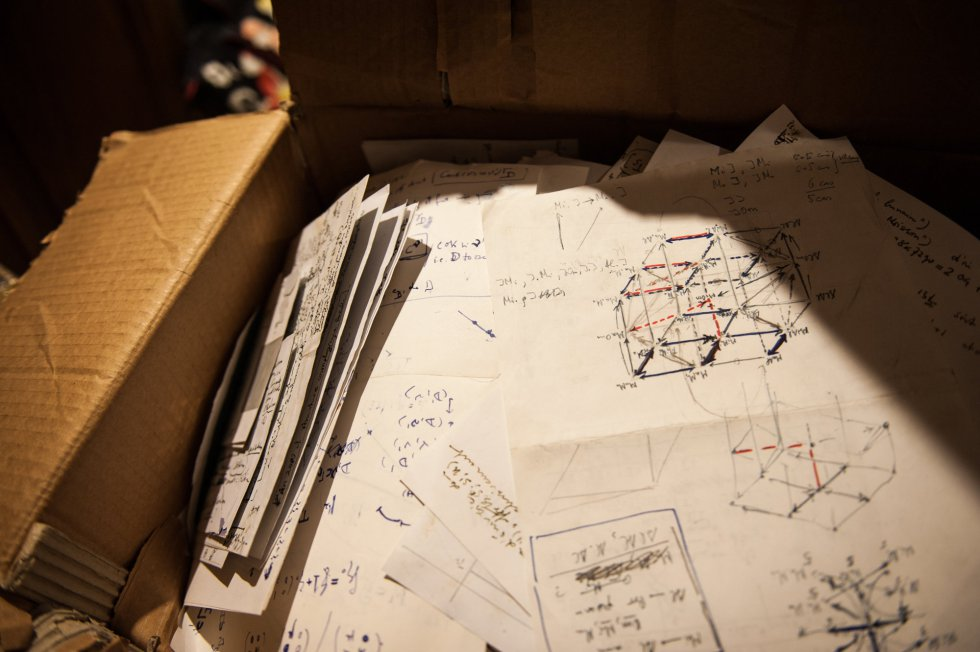Alexandre Grothendieck : une mathématique en cathédrale gothique, an article (in French) by Philippe Douroux in Le Monde, May 6th (behind paywall).

L’histoire étonnante des archives du mathématicien Alexandre Grothendieck, an article (in French) on France Inter by Mathieu Vidar, based on info from Philippe Douroux.

Les archives mystérieuses de Alexandre Grothendieck, a podcast of a broadcast on France Inter on June 10th. Interesting interview (in French) with Philippe Douroux and the French mathematician Etienne Ghys (with a guest appearance by Luc Illusie).

El enigmático legado de un genio de las matemáticas, an article (in Spanish) in El Pais, May 13th, with 8 photos of some of the Gribouillis. The two pictures in this post are taken from this article.

So, what’s the latest on the 70.000+ pages left by Grothendieck?

As far as i know, the Mormoiron part of the gribouillis is still at the University of Montpellier, and has been made available online at the Grothendieck archives.

The Lasserre part of the gribouillis is still in a cellar in Paris’ Saint-Germain-des-Prés, belonging to Jean-Bernard Gillot. The French national library cannot take possession of the notes before a financial agreement is reached with Grothendieck’s children (French law does not allow children to be disinherited).

And there’s a dispute about the price to be paid. The notes were estimated at 45.000 Euros, but some prefer to believe that they may be worth several millions of dollars.

It all depends on their mathematical content.

Unfortunately, pictures claimed to be of the Lasserre notes (such as the one above) are in fact from the Mormoiron/Montpellier notes, which do indeed contain interesting mathematics.

But, it is very unlikely that the Lasserre notes contain (math) surprises. Probably, most of them look like this one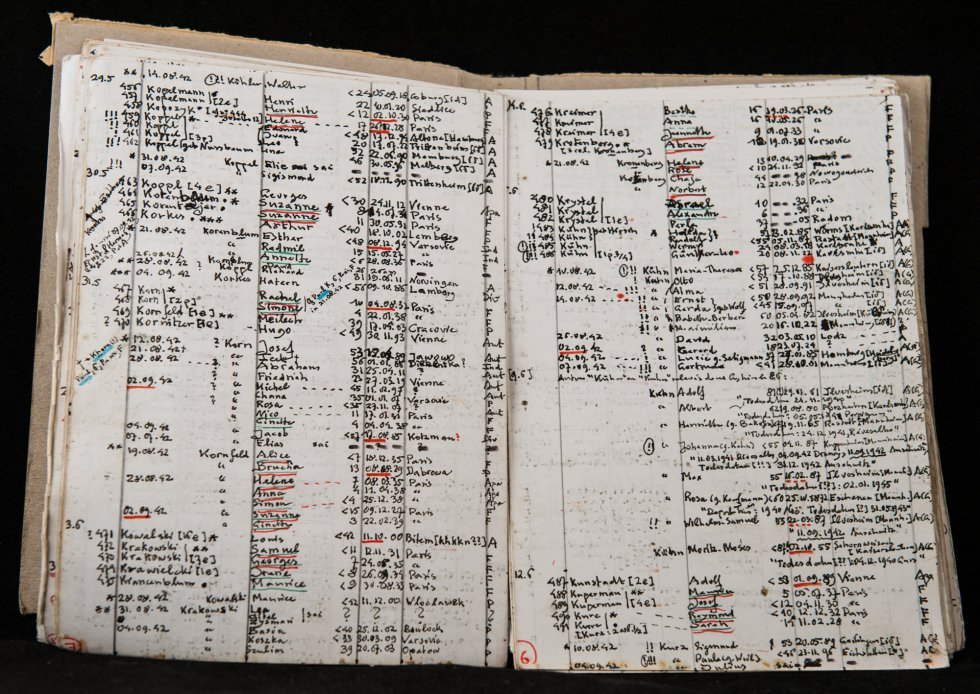endless lists of people deported by the Nazis to extermination camps in WW2.

Or, as Philippe Douroux is quoted in the El Pais piece: “I think it’s a treasure, maybe not a mathematical one, but a human one. It’s a descent into the hell of one the best organised brains in the world.”The film made by Catherine Aira and Yves Le Pestipon “Alexandre Grothendieck: On the Paths of a Genius” (on the quest for G’s last hideout in the French Pyrenees) can now be watched on YouTube (with English subtitles)

My Google+ account is going away on April 2, 2019, so i’ll try to rescue here some posts, in chronological order and around one theme. Here’s Grothendieck-stuff, part two.

March 18th, 2014

crowd-funding Grothendiecks biography?

+John Baez has a post out at the n-cat-cafe on Leila Schneps’s quest to raise \$6000 to translate Scharlau’s 3-volume biography of Grothendieck.

If you care to contribute : go here.

Lots of good stuff in volume 3 on Groths hippy/eco/weirdo years. I’ve plundered Scharlau’s text last year trying to pinpoint the location of Groths hideout in the French Pyrenees.

As far as i know, part 2 (the most interesting part on Groths mathematical years) is still under construction and will be compiled by the jolly group called the “Grothendieck circle”.

There’s a nice series of G-recollections out here (a.o. by Illusie, Karoubi, Cartier, Raynaud, Mumford, Hartshorne, Murre, Oort, Manin, Cartier).

I’m pretty sure Groth himself would prefer we’d try to get his Recoltes et Semailles translated into English, or La Clef des Songes.

November 18th, 2014

Grothendieck’s last hideout

The past ten days I’ve been up in the French mountains (without internet access), not that far from the Ariege, so I’m just now catching up with all (blog)posts related to Grothendieck’s death.

At our place, the morning of thursday november 13th was glorious!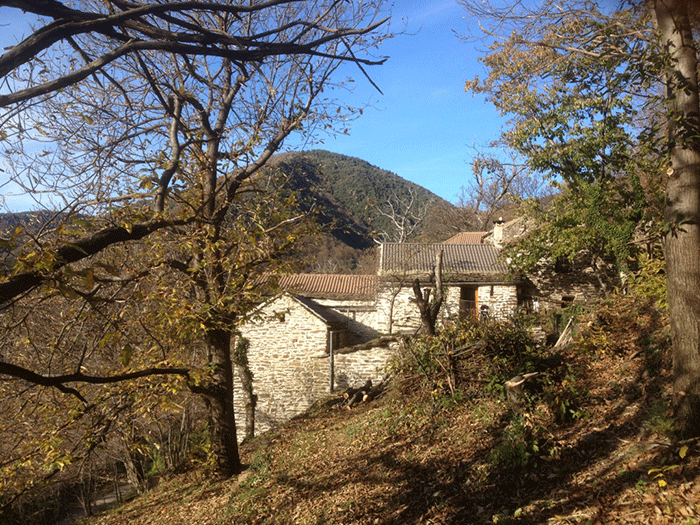Even though FranceInter kept telling horror stories about flooding in more southern departements, I can only hope that Grothendieck passed away in that morning sun.
About a year ago, on the occasion of Groth’s 85th birthday, I ran a series of posts on places where he used to live, ending with his last known hideout

At the time I didn’t include the precise location of his house, but now that pictures of it are in the French press I feel free to suggest (if you are interested to know where Grothendieck spend his later years) to point your Google-earth or Google-maps (in streetview!) to:
lat 43.068254  lon 1.169080

November 18th, 2014

Mormoiron and Lasserre acknowledge Grothendieck

In the series of post on Grothendieck-places I wrote a year ago (see here and links at the end) I tried to convince these French villages to update their Wikipedia page to acknowledge the existence of Grothendieck under the heading ‘Personnalités liées à la commune’, without much success.

Today it is nice to see that Lasserre added Grothendieck to their page:

“Alexandre Grothendieck (1928-2014), considéré comme un des plus grands mathématiciens du xxe siècle, y a vécu en quasi-ermite de 1990 à sa mort.”

Also Mormoiron, where Grothendieck lived in the 80ties (see picture below) has updated its page:

“Alexandre Grothendieck a habité temporairement à Mormoiron (“Les Aumettes”)”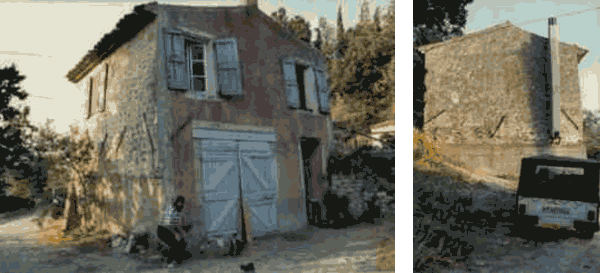French villages who still have to follow suit:
Vendargues
Massy
Olmet-et-Villecun

November 19th, 2014

+Leila Schneps invested a lot of time over the years setting up the Grothendieck Circle website.

Some material had to be removed a few years ago as per Groth’s request.

I’m sure many of you will be as thrilled as I was to get this message from Leila:

“I have already started modifying the Grothendieck circle website and it will of course eventually return completely.  Plus many things will be added, as we will now have access to Grothendieck’s correspondence and many other papers.”

Leila already began to update the site, for example there’s this new page on Groth’s life in Lasserre.

I understand Leila is traveling to Lasserre tomorrow, presumably for Grothendieck’s funeral. Hopefully she will eventually post something about it on the GrothendieckCircle (or, why not here on G+).

December 4th, 2014

Nicolas Bourbaki is temporarily resurrected to announce the death of Grothendieck in the French newspaper Le Monde.

You may recall that Bourbaki passed away on november 11th 1968, see +Peter Luschny’s post on his death announcement.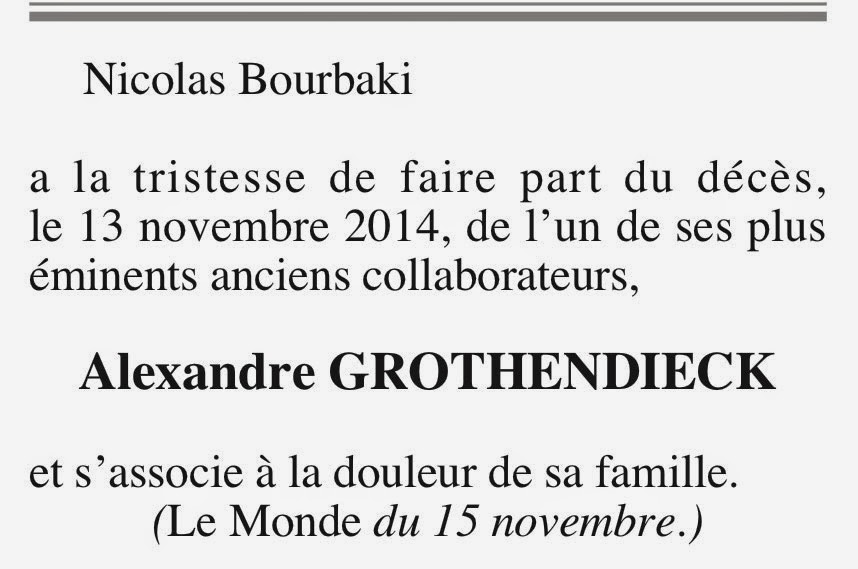December 6th, 2014

The ‘avis de décès’ released by Grothendieck’s family and friends, published in the local French newspaper ‘La Depeche’, on saturday november 15th.

It announces Grothendieck’s cremation, on november 17th at 11.30h in the village of Pamiers, bordering the ‘Camp du Vernet’, where Grothendieck’s father Sasha was imprisoned, before being deported to Auschwitz and murdered by the Nazis in 1942.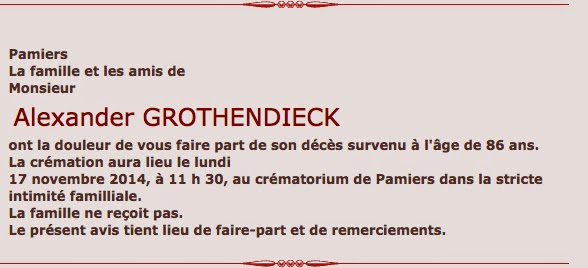June 12th, 2015

Grothendieck’s later writings

Next week there’s a Grothendieck conference at Montpellier. George Maltsiniotis will give a talk thursday afternoon with the  exciting title “Grothendieck’s manuscripts in Lasserre” (hat tip +Pieter Belmans ).

You may recall that G’s last hideout was in the Pyrenean village of Lasserre.

After a bit of sleuthing around I’ve heard some great news.

Grothendieck’s family have donated all of his later writings (apart from his correspondences and other family-related stuff) to the Bibliotheque Nationale. The BNF have expressed their intention of scanning all this material (thousands of pages it seems) and making them (eventually) available online!

Rumour has it that the donation consists of 41 large folders containing G’s reflections, kept in the form of a diary (a bit like ‘Clef des Songes’), on G’s usual suspects (evil, Satan, the cosmos), but 2 or 3 of these folders contain mathematics (of sorts).

Probably, Maltsiniotis will give a preview on this material. To anyone lucky enough to be able to go down south next week and to attend his talk, please keep me in the loop…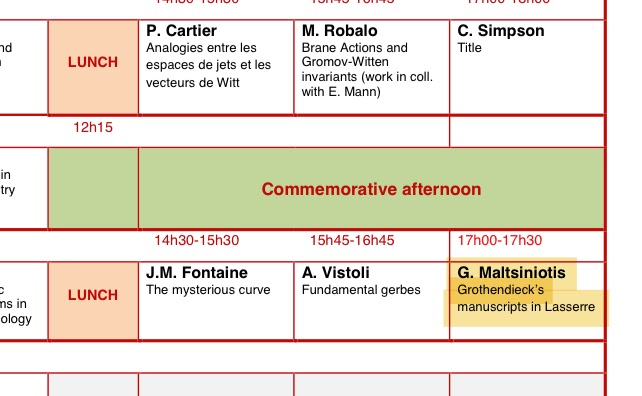June 19th, 2015

Maltsiniotis’ talk on Grothendieck’s Lasserre-gribouillis

Yesterday, George Maltsiniotis gave a talk at the Gothendieck conference in Montpellier with title “Grothendieck’s manuscripts in Lasserre”.

This morning, +David Roberts  asked for more information on its content, and earlier i gave a short reply on what i learned, but perhaps this matter deserves a more careful write-up.

+Damien Calaque  attended George’s talk and all info below is based on his recollections. Damien stresses that he didn’t take notes so there might be minor errors in the titles and order of the parts mentioned below.

EDIT: based on info i got from +Pieter Belmans  in the comments below (followed up by the picture he got via +Adeel Khan  taken by Edouard Balzin) i’ve corrected the order and added additional info.

The talk was videotaped and should become public soon.

As i mentioned last week Grothendieck’s family has handed over all non-family related material to the Bibliotheque Nationale. Two days ago, Le Monde wrote that the legacy consists of some 50.000 pages.

Maltsiniotis insisted that the BNF wants to make these notes available to the academic community, after they made an inventory (which may take some time).

I guess from the blackboard-picture i got from Pieter, the person responsible at the BNF is Isabelle le Masme de Chermont.

The Lasserre-griboillis themselves consists of 5 parts:

1. Géométrie élémentaire schématique. (August 1992)

2. Structure de la psyché. (12/10/1992-28/09/1993) 3600 pages
This one is about some combinatorics of oriented graphs with extra-structure (part of the structure are successor and predecessor operators on the set of arrows).

3. Psyché et structures (26/03/93-20/06/93) 700 pages
This one is non-mathematical.

4. Maxwell equations.
Maltsiniotis mentioned that he was surprised to see that there was at best one mathematics book in G’s home, but plenty of physics books.

5. Le problème du mal. (1993-1998)
This one is huge (30.000 pages) and is non-mathematical.

Note that also the Mormoiron-gribouillis will be made public by the University of Montpellier, or if you prefer video.

Finally, is the photo below what you think it is? Yep!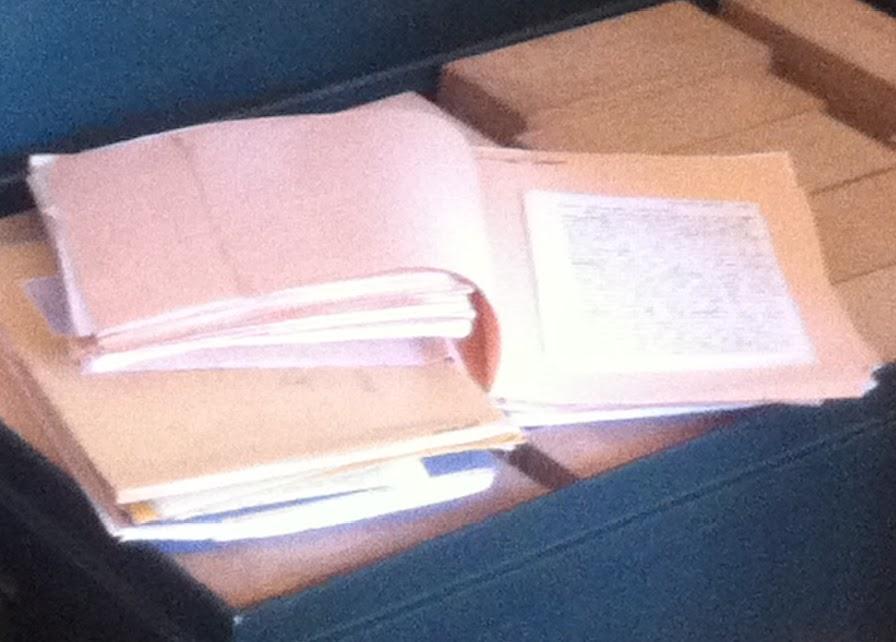January 20th, 2016

where are the videos of the Grothendieck conference?

Mid june 2015 a conference “Mathematics of the 21st century: the vision of Alexander Grothendieck” was held in Montpellier. In a comment to a post here on Maltsiniotis’ talk i mentioned that most of the talks were video-taped and that they would soon be made public.

When they failed to surface on the Montpellier website, i asked +Damien Calaque  for more information. Some months ago Damien told me the strange (and worrying) tale of their fate.

At that moment Damien was in a process of trying to recover the videos. Two weeks ago he told me things were looking good, so i now feel free to post about it.

Michael Wright is the head of the Archive for Mathematical Sciences & Philosophy. He arranged with the organizers of the conference that he would send someone over to video-tape the lectures and that he would make them available on his Archive. He also promised to send a copy of the videos to Montpellier, but he never did. Nor did the tapes appear on his site.

Damien Calague emailed Wright asking for more information and eventually got a reply. It appears that Wright will not be able to edit the videos nor put them online in a reasonable time.

They agreed that Damien would send him a large capacity USB-drive. Wright would copy the videos on it and send it back. Damien will arrange for the videos to be edited and the University of Montpellier will put them online. Hopefully everything will work out smoothly.

So please keep an eye on the website of l’Institut Montpelliérain Alexander Grothendieck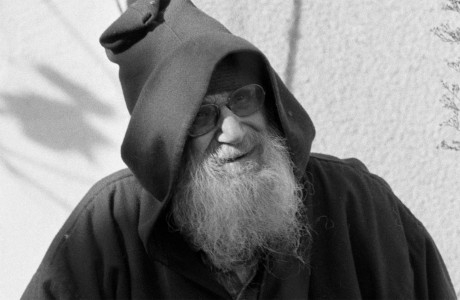May 6th, 2017

Grothendieck’s Montpellier notes will hit the net May 10th

At last there is an agreement between the university at Montpellier and Grothendieck’s children to release the ‘Montpellier gribouillis’ (about 28000 pages will hit the net soon).
Another 65000 pages, found at Lasserre after Grothendieck’s death, might one day end up at the IHES or the Bibliotheque Nationale.
If you are interested in the history of Grothendieck’s notes, there is this old post on my blog.

(h/t Theo Raedschelders for the Liberation link)

May 11th, 2017

Buy a Grothendieck painting to get the Lasserre notes online!

As of yesterday, most of Grothendieck’s Montpellier notes are freely available at this site.

There’s much to say about the presentation (eg. It is not possible to link directly to a given page/article, it is scanned at only 400 dpi etc. etc.) but hey, here they are at last, for everyone to study.

By far the most colourful (in my first browsing of the archive) is cote No. 154, on ‘systeme de pseudo-droites’. You can download it in full (a mere 173 Mb).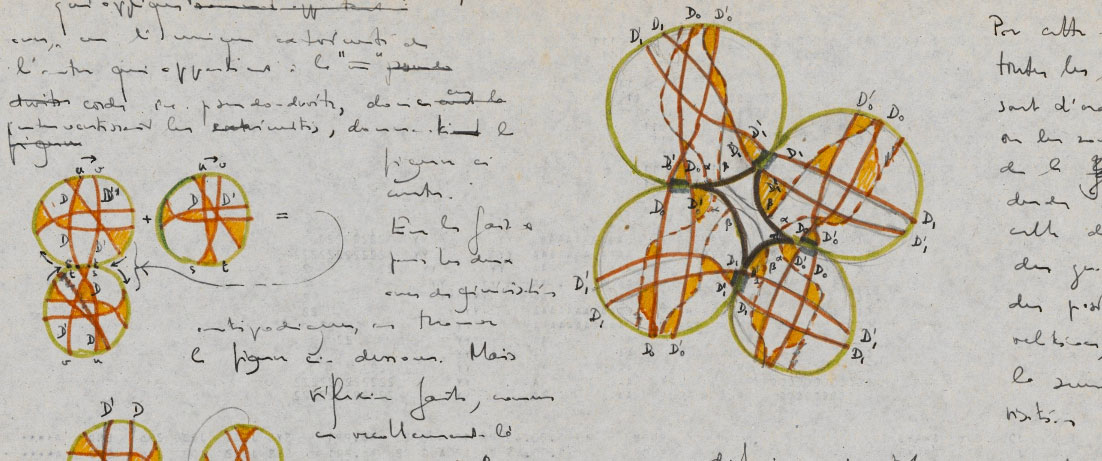As you know, the Montpellier notes are only a fraction of the material Grothendieck left behind. By far the largest (though probably not the most interesting, mathematically) are the Lasserre notes, which to the best of my knowledge are in the care of a Parisian bookseller.

Here’s an idea:
almost every page of No. 154 (written on ancient computer-output) looks like a painting. No doubt, most math departments in the world would love to acquire one framed page of it. Perhaps this can raise enough money to safeguard the Lasserre notes…

July 13th, 2017

le Tour de France in Grothendieck’s backyard

If you want to see the scenery Grothendieck enjoyed in his later years, watch the Tour de France tomorrow.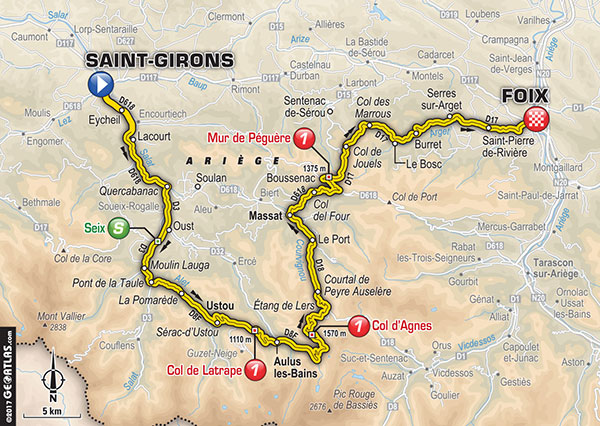It starts in Saint-Girons where he went to the weekly market (and died in hospital, november 13th 2014), ending in Foix with 3 category 1 climbs along the way (familiar to anyone familiar with Julia Stagg’s expat-lit set at ‘Fogas’ or you can read my own post on Fogas).

It will not pass through Lasserre (where G spend the final 20 years of his life) which is just to the north of Saint-Girons.